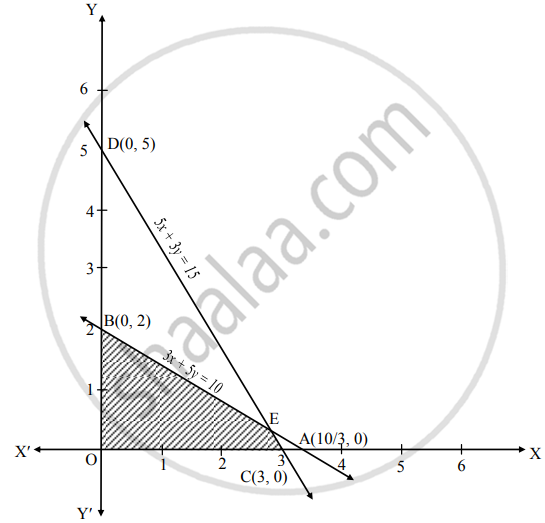Advertisement Remove all ads

# Solve the following problem : Maximize Z = 6x + 10y Subject to 3x + 5y ≤ 10, 5x + 3y ≤ 15, x ≥ 0, y≥0 - Mathematics and Statistics

Graph

Solve the following problem :

Maximize Z = 6x + 10y Subject to 3x + 5y ≤ 10, 5x + 3y ≤ 15, x ≥ 0, y≥0

Advertisement Remove all ads

#### Solution

To find the graphical solution, construct the table as follows:

 Inequation equation Double intercept form Points (x1, x2) Points (x1, x2) 3x + 5y ≤ 10 3x + 5y = 10 x/(10/3) + y/(2) = 1 A (10/3, 0) B (0, 2) 3(0) + 5(0) ≤ 10∴ 0 ≤ 10∴ Origin-side 5x + 3y ≤ 15 5x + 3y = 15 x/(3) + y/(5) = 1 C (3, 0) D (0, 5) 5(0) + 3(0) ≤ 15∴ 0 ≤ 15∴ Origin-side x ≥ 0 x = 0 – R.H.S. of Y-axis y ≥ 0 y = 0 above X-axisThe shaded portion OCEB is the feasible region.
Whose vertices are O(0, 0), C(3, 0), E and B(0, 2)
E is the point of intersection of lines
3x + 5y = 10 …(i)
5x + 3y = 15 …(ii)
∴ By 5(i) – 3(ii), we get
15x + 25y = 50
15x +   9y = 45
–        –       –
16y = 5

∴ y = (5)/(16)

Substituting y = (5)/(16) in (i), we get

3x+ 5 xx (5)/(16) = 10

∴ x = (45)/(16)

∴ E(45/16, 5/16)
Here, the objective function is Z = 6x + 10y
Now, we will find minimum value of Z as follows:

 Feasible points The value of Z = 6x + 10y O (0, 0) Z = 6(0) + 10(0) = 0 C (3, 0) Z = 6(3) + 10(0) = 18 E(5/16, 45/16) Z = 6(45/16) + 10(5/16) = 20 B (0, 2) Z = 6(0) + 10(2) = 20

∴ Z has maximum value 20 at all points on the line 3x + 5y = 10 between B (0, 2) and E(45/16, 5/16)

∴ There are infinite number of optimum solutions of the given LPP.

Is there an error in this question or solution?
Advertisement Remove all ads

#### APPEARS IN

Balbharati Mathematics and Statistics 2 (Commerce) 12th Standard HSC Maharashtra State Board
Chapter 6 Linear Programming
Miscellaneous Exercise 6 | Q 4.03 | Page 104
Advertisement Remove all ads
Advertisement Remove all ads
Share
Notifications

View all notifications

Forgot password?